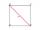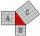# Trio 2

Decide whether trio of numbers is the side of a right triangle: 26,24,10.

Result

#### Solution:We would be pleased if you find an error in the word problem, spelling mistakes, or inaccuracies and send it to us. Thank you!Tips to related online calculators
Pythagorean theorem is the base for the right triangle calculator.

#### You need to know the following knowledge to solve this word math problem:

We encourage you to watch this tutorial video on this math problem:

## Next similar math problems:

• Reverse Pythagorean theoremGiven are lengths of the sides of the triangles. Decide which one is rectangular: Δ ABC: 77 dm, 85 dm, 36 dm ? Δ DEF: 55 dm, 82 dm, 61 dm ? Δ GHI: 24 mm, 25 mm, 7 mm ? Δ JKL: 32 dm, 51 dm, 82 dm ? Δ MNO: 51 dm, 45 dm, 24 dm ?
• Height UTHow long is height in the equilateral triangle with a side b = 43?
• Square 2Points D[10,-8] and B[4,5] are opposed vertices of the square ABCD. Calculate area of the square ABCD.
• Is right?Is triangle with sides 51, 56 and 77 right triangle?Ladder 10 meters long is staying against the wall so that its bottom edge is 6 meters away from the wall. What height reaches ladder?
• Two diagonalsThe rhombus has a side length 12 cm and length of one diagonal 21 cm. What is the length of the second diagonal?
• HypotenuseCalculate the length of the hypotenuse of a right triangle with a catheti 71 cm and 49 cm long.Ladder 8 m long is leaning against the wall. Its foot is 1 m away from the wall. In which height ladder touches the wall?
• SquareCalculate the side of a square with a diagonal measurement 10 cm.
• SquareRectangular square has side lengths 183 and 244 meters. How many meters will measure the path that leads straight diagonally from one corner to the other?
• Right isoscelesCalculate area of the isosceles right triangle which perimeter is 41 cm.
• Triangular pyramidWhat is the volume of a regular triangular pyramid with a side 3 cm long?
• Chord ABWhat is the length of the chord AB if its distance from the center S of the circle k(S, 92 cm) is 10 cm?
• Is right?Determine whether the triangle with legs (catheti) 19.5 cm and 26 cm and length of the hypotenuse 32.5 cm is rectangular?
• Floating barrelBarrel (cylinder shape) floats on water, top of barrel is 8 dm above water and the width of surfaced barrel part is 23 dm. Barrel length is 24 dm. Calculate the volume of the barrel.
• AP RT triangleThe length of the sides of a right triangle form an arithmetic progression, longer leg is 24 cm long. What are the perimeter and area?
• GardenArea of a square garden is 6/4 of triangle garden with sides 56 m, 35 m, and 35 m. How many meters of fencing need to fence a square garden?